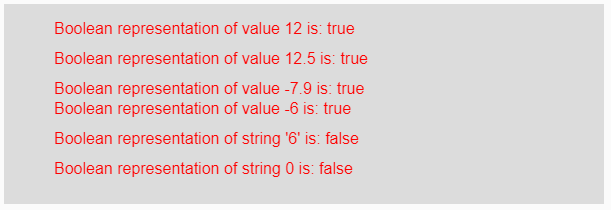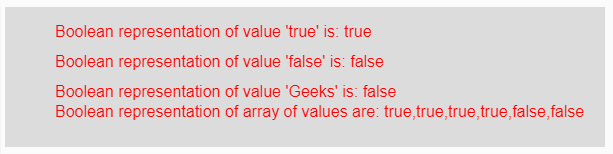# p5.js | boolean() function

• Last Updated : 12 Apr, 2019

The boolean() function in p5.js is used to convert the given string and number value into its boolean representation.

Syntax:

Hey geek! The constant emerging technologies in the world of web development always keeps the excitement for this subject through the roof. But before you tackle the big projects, we suggest you start by learning the basics. Kickstart your web development journey by learning JS concepts with our JavaScript Course. Now at it's lowest price ever!

`boolean(Value)`

Parameters: This function accepts single parameter Value which are to be converted into its boolean representation. This value might be integer, float, string, boolean, negative or positive value and array of values.

Return Value: It returns the converted boolean representation.

Below program illustrates the boolean() function in p5.js:

Example 1: This example uses boolean() function to convert input value into its boolean value.

 `function` `setup() { ``  ` `    ``// Creating Canvas size``    ``createCanvas(600, 200); ``} ``  ` `function` `draw() { ``      ` `    ``// Set the background color ``    ``background(220); ``    ` `    ``// Initializing some values``    ``let Value1 = 12;``    ``let Value2 = 12.5;``    ``let Value3 = -7.9;``    ``let Value4 = -6; ``    ``let Value5 = ``"6"``;``    ``let Value6 = 0;``    ` `    ``// Calling to boolean() function.``    ``let A = boolean(Value1);``    ``let B = boolean(Value2);``    ``let C = boolean(Value3);``    ``let D = boolean(Value4);``    ``let E = boolean(Value5);``    ``let F = boolean(Value6);``      ` `    ``// Set the size of text ``    ``textSize(16); ``      ` `    ``// Set the text color ``    ``fill(color(``'red'``)); ``    ` `    ``// Getting boolean representation``    ``text(``"Boolean representation of value 12 is: "` `+ A, 50, 30);``    ``text(``"Boolean representation of value 12.5 is: "` `+ B, 50, 60);``    ``text(``"Boolean representation of value -7.9 is: "` `+ C, 50, 90);``    ``text(``"Boolean representation of value -6 is: "` `+ D, 50, 110);``    ``text(``"Boolean representation of string '6' is: "` `+ E, 50, 140);``    ``text(``"Boolean representation of string 0 is: "` `+ F, 50, 170);``} `

Output:Example 2: This example uses boolean() function to convert input value into its boolean value.

 `function` `setup() { ``  ` `    ``// Creating Canvas size``    ``createCanvas(600, 140); ``} ``  ` `function` `draw() { ``      ` `    ``// Set the background color ``    ``background(220); ``    ` `    ``// Initializing some values``    ``let Value1 = ``true``;``    ``let Value2 = ``false``;``    ``let Value3 = ``"Geeks"``;``    ``let Value4 = [12, 3.6, -9.8, ``"true"``, ``false``, ``"Geeks"``];``    ` `    ``// Calling to boolean() function.``    ``let A = boolean(Value1);``    ``let B = boolean(Value2);``    ``let C = boolean(Value3);``    ``let D = boolean(Value4);``      ` `    ``// Set the size of text ``    ``textSize(16); ``      ` `    ``// Set the text color ``    ``fill(color(``'red'``)); ``    ` `    ``// Getting boolean representation``    ``text(``"Boolean representation of value 'true' is: "` `+ A, 50, 30);``    ``text(``"Boolean representation of value 'false' is: "` `+ B, 50, 60);``    ``text(``"Boolean representation of value 'Geeks' is: "` `+ C, 50, 90);``    ``text(``"Boolean representation of array of values are: "` `+ D, 50, 110);``}   `

Output:Note: From the above examples, If the parameter is non-zero value then it returns true and for strings, only “true” string returns true otherwise it returns false output for any other string.

Reference: https://p5js.org/reference/#/p5/boolean

My Personal Notes arrow_drop_up